#### Number of problems found: 2591

• A large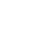A large gear will be used to turn a smaller gear. The large gear will make 75 revolutions per minute. The smaller gear must make 384 revolutions per minute. Find the smallest number of teeth each gear could have. [Hint: Use either GCF or LCM. ]
• Photocopier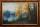A photocopier enlarges a picture in the ratio 7:4. How many times will a picture of size 6cm by 4cm be enlarged to fit on a 30cm by 20 cm page?
• ParkingAt a building parking, 245 spaces are for cars, 56 are for vans and the rest are for buses. If 14% of all parking spaces are for buses, how many parking spaces are there in the building? How many spaces are for buses?
• Lookout towerHow high is the lookout tower? If each step was 3 cm lower, there would be 60 more of them on the lookout tower. If it was 3 cm higher again, it would be 40 less than it is now.
• Internal anglesFind the internal angles of the triangle ABC if the angle at the vertex C is twice the angle at the B and the angle at the vertex B is 4 degrees smaller than the angle at the vertex A.
• Right angled triangle 3Side b = 1.5, hypotenuse angle A = 70 degrees, Angle B = 20 degrees. Find its unknown sides length.
• Electric workCalculate the work done by the electric forces passing the current of 0.2 A through the bulb in 10 minutes if the bulb is connected to a 230 V power supply.
• Tulips and daffodils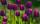Two hundred twenty tulips and daffodils are planted in the flowerbed. One-third of all tulips and one-sixth of all daffodils equals the number of all tulips. How many tulips and how many daffodils?
• Tractors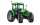Wheel and belt tractors each day plow 8 ha of fields. For plow 76 hectares, the wheel would have to work for 12 days and 8 days with the belt tractor together. How many hectares do each day of each tractor?
• Square and circlesThe square in the picture has a side length of a = 20 cm. Circular arcs have centers at the vertices of the square. Calculate the areas of the colored unit. Express area using side a.
• The observatoryThe dome of the hemisphere-shaped observatory is 5.4 meters high. How many square meters of sheet metal needs to be covered to cover it, and 15 percent must be added to the minimum amount due to joints and waste?
• Parallelogram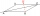Rhomboid (parallelogram) has a longer side of 50 cm long. The size of its one height is four times the size of its second height. Calculate the length of the shorter side of this rhomboid in the centimeters.
• Summer tiresThree tire servants have to change the summer tires on 6 cars in 2 hours. Mark's replacement would take 4.5 hours, Jirka would do it in 3 hours and 10 minutes, and Honza in 4 hours. Will they be able to replace all tires at the desired time?
• Mba studiumAt MBA school, fourth-year students can choose from three optional subjects: a) mathematical methods, b) social interaction, c) management Each student studies one of these subjects. The mathematical methods studied 28 students, the social interaction 27
• Free space in the gardenThe grandfather's free space in the garden was in the shape of a rectangular triangle with 5 meters and 12 meters in length. He decided to divide it into two parts and the height of the hypotenuse. For the smaller part creates a rock garden, for the largeThe ladder has a length of 3 m and is leaning against the wall, and its inclination to the wall is 45°. How high does it reach?
• In aIn a triangle, the aspect ratio a: c is 3: 2 and a: b is 5: 4. The perimeter of the triangle is 74cm. Calculate the lengths of the individual sides.
• Surface of the cylinderCalculate the surface of the cylinder for which the shell area is Spl = 20 cm2 and the height v = 3.5 cm
• Two friendsPeter can do all his work himself in 6 hours. Martin can do the same work himself in 8 hours. Peter worked first and then replaced by Martin. Whole work was done in 6.5 hours. Calculate how long Peter worked before replaced by Martin.The radius of the sphere we reduce by 1/3 of the original radius. How much percent does the volume and surface of the sphere change?